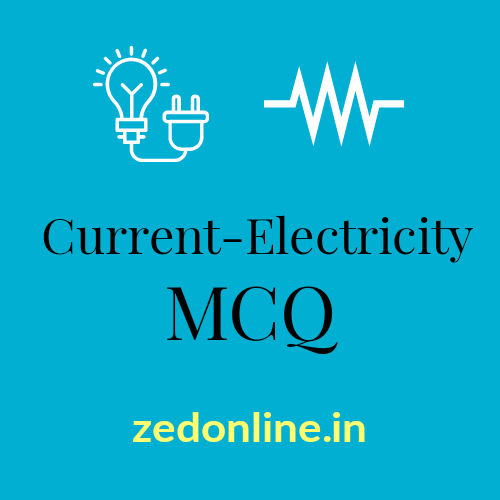Most important Madhyamik Physics MCQs with answers from the chapter Current Electricity. Learn the MCQ from Current-Electricity chapter to secure good marks un class 10. Also Question asked in the previous madhyamik examination are included in this series.

## 1. In household connection, the fuse is always connected with the –

a. Live wire
b. Neutral wire
c. Earth wire
d. Can be connected to any wire of the above

a. Ampere
c. Coulomb
d. Oersted
Ans: d. Oersted

a. Doubled
b. Four times
c. Half
d. Four times
Ans: a. Doubled

## 4.The SI unit of electric power and the correct relation relating it is –

a. watt, watt = coulomb x time
b. watt, watt = coulomb x volt
c. watt, watt = ampere x volt
d. joule, joule = coulomb x volt
Ans: c. watt, watt = ampere x volt

a. 16 A
b. 6 A
c. 4 A
d. 1.6 A
Ans: c. 4 A

## 6.Emf of an electrical cell is the –

a. Potential appear across the external load
b. Potential drop across its internal resistance
c. Difference of potential at its two ends when it is open circuited
d. None of these
Ans: c. Difference of potential at its two ends when it is open circuited.

## 7.According to thumb rule –

a. If the thumb of our any hand indicates the direction of current, then wrapped fingers will represent
circular magnetic field around the current
b. If the thumb of our left hand indicates the direction of current, then wrapped fingers will represent
circular magnetic field around the current
c. If the thumb of our left hand indicates the direction of magnetic field, then wrapped fingers will
represent direction of the current
d. If the thumb of our right hand indicates the direction of current, then wrapped fingers will represent
circular magnetic field around the current
Ans: d. If the thumb of our right hand indicates the direction of current, then wrapped fingers will represent circular magnetic field around the current.

## 8. If a person is assumed to swim along the direction of current and faces a magnetic needle, then

a. The N pole of the needle will deflect towards his right hand
b. The S pole of the needle will deflect towards his right hand
c. The S pole of the needle will deflect towards his left hand
d. None of these
Ans: b. The S pole of the needle will deflect towards his right hand.

## 9.In Fleming’s left hand rule, thumb of the left hand indicates

a. Magnetic field
b. Direction of current
c. Deflection of magnetic needle
d. None of these.
Ans: d. None of these.

## 10.Electric motors work under the principle of

a. Electromagnetic induction
b. Fleming’s right hand rule
c. Lenz’s law
d. Conversion of electrical energy to mechanical energy
Ans: d. Conversion of electrical energy to mechanical energy

a. Ampere
c. Coulomb
d. Oersted
Ans: d. Oersted.

## 12.Chose the correct relation –

a. joule = coulomb x volt
b. joule = ampere x volt
c. watt = joule x sec
d. watt = volt x coulomb
Ans: a. Joule = coulomb x volt

## 13.The commercial unit of electric energy is B.O.T. 1 B.O.T =

a. 1 Watt-hr
b. 1000watt-sec
c. 100 watt-hr
d. 1kwatt-hr
Ans: d. 1kwatt-hr

## 14.According to Ohm’s law –

a. Current in a conductor is directly proportional to the resistance
b. Current in a conductor is directly proportional to the potential
c. Current in a conductor is directly proportional to the potential difference
d. Current in a conductor is inversely proportional to the potential difference
Ans: c. Current in a conductor is directly proportional to the potential difference.

## 15.If a conductor is heated to increase its temperature, then its resistance will –

a. Increase
b. Decrease
c. Remain same as resistance does not depend on temperature
d. First increase and then decrease
Ans: a. Increase

## 16.The resistance of a conducting slab depends on –

a. Length of the conductor
b. Area of cross section of the conductor
c. Nature of the conductor
d. All of these
Ans: d. All of these

a. ampere
b.Volt.
c. Watt
d.ohm

a.volt
b. watt
c.ampere
d. ohm

a. volt
b. ampere
c. coulomb
d.ohm

a.coulomb
b.ampere
c.volt
d. ohm

a. ohm-m
b.ohm-cm
c.ohm
d.ohm-ft

a. 6 coulomb
b. 60 coulomb
c.5 coulomb
d. 15 coulomb

a. Q=WV
b. Q=V/W
c. Q= V/W2
d. Q=W/V

## 24. Which of the physical quantity represent ampere. (MP-2017)

a. coulomb-second
b. volt-ohm-1
c. volt-ohm
d. volt-1 ohm

## 25. Resistivity of which of the following decreases with increase in temperature? (MP-2017)

a.conductor
b.semiconductor
c. super conductor
d.Insulator